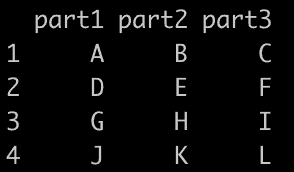# How to Use separate() Function in R

The separate() function from the tidyr package in R is “used to separate a single data frame or table column into multiple columns.”

## Syntax

``````separate(
df,
col,
into,
sep = "[^[:alnum:]]+",
remove = TRUE,
convert = FALSE
)
``````

## Parameters

1. df: It is the data frame.
2. column: It is a column that is to be separated.
3. into: The names of columns used for the data to be separated.
4. sep: This is the value to separate the data. default = _[^[:alnum:]]+_, regular expression.
5. remove: If set to TRUE, remove input column(s) from the output data frame. Default = TRUE.
6. convert: This is used for datatype conversions. Default = FALSE.

## Example 1: Separate Column into Two Columns

``````library(tidyr)

df <- data.frame(
name = c("John_Doe", "Jane", "Alex_Brown_Jr")
)

df_separated <- separate(df,
col = "name", into = c("first_name", "last_name"),
sep = "_", extra = "merge", fill = "right"
)

print(df_separated)``````

Output

``````   first_name   last_name
1    John          Doe
2    Jane          <NA>
3    Alex         Brown_Jr``````

## Example 2: Separate Column into More Than Two Columns

The separate() function from the tidyr package in R can be used to split a column into more than two columns. The process is similar to splitting into two columns; you just provide more column names in the argument.

``````library(tidyr)

df <- data.frame(column_to_split = c("A_B_C", "D_E_F", "G_H_I", "J_K_L"))

df_separated <- separate(df,
col = "column_to_split",
into = c("part1", "part2", "part3"),
sep = "_"
)

print(df_separated)
``````

OutputThat’s it!